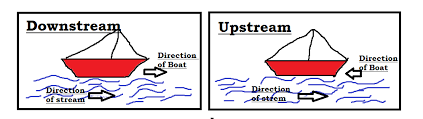# Boats and Streams Tricks & Shortcuts for RRB & Other Competitive ExamsBoats and Streams. Before going to the topic, Let us know what is Downstream and what is Upstream. Because these concepts are the heart of Boats and Streams.
Downstream
In water,the direction along the stream is called downstream. I mean, If a boat or a swimmer swims in the same direction as the stream, then it is called downstream. Obviously the boat or swimmer require less efforts to travel using downstream. Because the stream itself helps the objects to move.
Remember, as the object moves along with the water, the stream helps the object.
So, the down stream speed (DS) is
DS =  U+V
where U is the speed of the object in the still (calm) water
V is the speed of the water.Upstream
If the boat or the swimmer is swimming in the opposite direction in which the stream is passing is called upstream. In simple words, the direction against the stream is called upstream.
Remember, as the object moves against the water pushes the object in opposite direction.
So, the upstream speed (US) is
US = U-V
where U is the speed of the object in the still (calm) water
V is the speed of the water.

Important Formulas on Boats and Streams

Let us assume that the speed of the boat in still water is U km/hr and the speed of stream is V km/hr then, as mentioned above :

Speed downstream = (u+v)km/hr

Speed upstream = (u-v)km/hr
If the speed downstream is a km/hr and the speed upstream is b km/hr then :
Speed in still water = 1/2(a+b) km/hr
Rate of stream = 1/2(a-b)km/hr
Problems on Boats and Streams – Model :: 1

1)A man can row upstream at 8kmph and downstream at 10kmph. Find the man’s rate in still water and the rate of current.
Solution : Rate in still water = 1/2 (10+8)km/hr = 9km/hr
Rate of current = 1/2(10-8)km/hr =1km/hr
2)A boat can travel with a speed of 12km/hr in still water . If the speed of the stream is 5km/hr , find the time taken by boat to go 68km downstream.
solution : Speed downstream = (12+5)km/hr  = 17 km/hr
Time taken to travel 68km downstream = 68/17hrs  =4hrs
3)If a man rows at the rate of 5kmph in still water and his rate against the current is 3.5kmph, then man’s rate along the current is ?
solutinLet us consider the rate along the current be x kmph

Then, 1/2(x+3.5) = 5  or X= 6.5 Kmph
4)A boat covers a certain distance downstream in 1 hour, while it comes back  in 3/2 hours. If the speed of the stream is 3kmph, what is the speed of the boaat in still water ?
Solution : Let the speed of the boat in still water be x kmph.
Then speed downstream = (X+3)kmph
Speed upstraem = (x-3)kmph
Therefore (X+3)*1 = (x-3)*3/2
2x+6=3x-9  => X= 15kmph
5)A speed of a boat in still water is 10 km/hr. If it can travel 26km downstream and 14km upstream in the same time , the speed of the stream is ?
solution : let the speed of the stream be x km/hr. then,
Speed downstream=(10+x)km/hr,
speed upstream= (10-x)km/hr
Therefore  26/(10+x)= 14/(10-x)
260-26x= 140+14x  => 40x=120
x=3km/hr

Problems on Boats and Streams – Model : 2

6)The speed of the boat in still water is 10km/hr and the rate of the current is 3 km/hr. The distance travelled downstream in 12 minutes is ?
solution : Speed downstream =(10+3)kmph= 13kmph
Distance travelled = 13*12/60 = 2.4 kmph
7)what is the speed of the boat in still water ?
i)The speed downstream of the boat is twice the speed upstream.
ii)The sum of the speeds of the boat , upstream and downstream is 12kmph
solution: Let the speed of the boat be x km/hr . Then
i) Speed downstream = 2x km/hr
ii)Says, the speed of the boat in still water = (1/2*12) = 6km /hr
8)A man can row upstream at 5kmph and downstream at 15kmph .The speed of the stream is ?
Solution :    Speed of the stream = 1/2 (15-5) = 5kmph
9)A boat takes a total time three hours to travel downstreams from P to Q and the upstream back from Q to P. what is the speed of boat in still water ?
i)The speed of the river current is 1 km per hour
ii)The distance between P and Q is 4 km.
solution :  i) The speed of the current = 1km/hr
ii)PO =4km
let the speed of the boat in still water be x km/hr
4/(x+1) +4/(x-1) =3
10)A man takes twice long to row a distance against the stream as to row the same distance in favour of the stream .the ratio of the speed of the boat in still water is ?
solution : Let man’s upstream be xkmph . then . his rate downstream =3x km/hr
(Speed in still water) : (Speed of stream ) = (2x+x/2) : (2x-x/2) =3x/2 : x/2
=> 3:1

#### క్రిస్ సాఫ్ట్వేర్ ఇంజనీర్లు

error: Content is protected !!